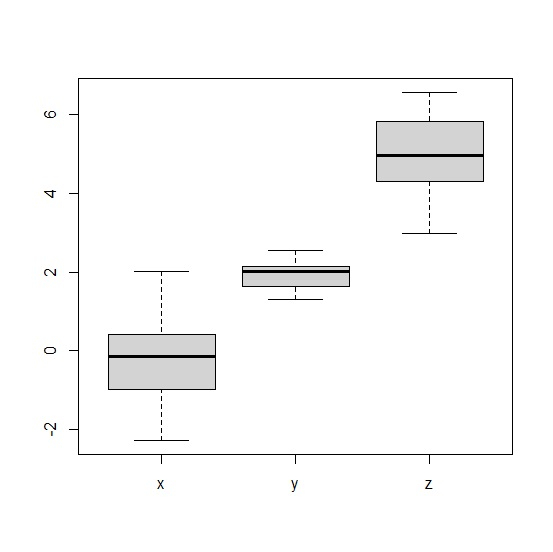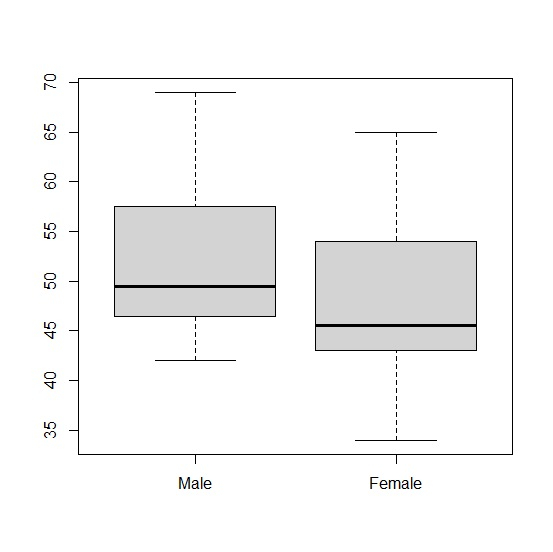# How to extract statistical summary from boxplot in R?

To extract statistical summary from boxplot we can use stats function with delta operator. For example, if we have a data frame called df that contains 5 columns then the boxplot for each column can be created by using the command boxplot(df) and if we want to extract the statistical summary from this boxplot then boxplot(df)$stats can be used. Consider the below data frame − ## Example Live Demo df<-data.frame(x=rnorm(20),y=rnorm(20,2,0.35),z=rnorm(20,5,0.87)) df ## Output  x y z 1 0.42464003 2.174201 5.867968 2 -2.26742343 2.015934 5.016444 3 0.53905022 2.030312 5.317082 4 0.45316334 2.544108 6.561526 5 -0.20622226 2.523544 5.800500 6 -1.04035346 2.159960 4.796673 7 -1.55531189 2.079003 3.412160 8 -1.49530817 2.009581 4.052736 9 0.39796949 1.575125 5.328166 10 -0.33719074 1.667450 4.302307 11 -0.02416107 2.100736 2.979569 12 -0.19110426 2.148660 4.439635 13 0.12629817 1.593884 5.854684 14 -0.89502785 1.564742 3.298984 15 0.03870768 1.791996 4.968653 16 -0.24417567 1.315945 4.748624 17 0.44633643 2.128895 4.301884 18 2.01719942 2.139194 6.006298 19 -1.23681599 1.783813 6.557920 20 -0.10166870 1.502973 4.939246 Creating boxplot for columns in df − ## Example boxplot(df) ## OutputExtracting the statistical summary from boxplot of df − ## Example Summary<-boxplot(df)$stats
colnames(Summary)<-c("x","y","z")
rownames(Summary)<-c("Min","First Quartile","Median","Third Quartile","Maximum")
Summary

## Output

                   x          y         z
Min            -2.2674234  1.315945  2.979569
First Quartile -0.9676907  1.630667  4.302095
Median         -0.1463865  2.023123  4.953950
Third Quartile  0.4113048  2.143927  5.827592
Maximum         2.0171994  2.544108  6.561526

## Example

Live Demo

Gender_data<-data.frame(Male=rpois(20,50),Female=rpois(20,50))
Gender_data

## Output

   Male Female
1  56   34
2  48   58
3  48   55
4  50   37
5  47   53
6  56   46
7  56   50
8  48   42
9  44   37
10 59   54
11 69   44
12 53   43
13 66   43
14 42   45
15 49   54
16 45   45
17 46   45
18 42   61
19 68   46
20 60   65

## Example

Summary<-boxplot(Gender_data)

## OutputExtracting the statistical summary from boxplot of df −

## Example

Summary<-boxplot(Gender_data)\$stats
colnames(Summary)<-c("Male","Female")
rownames(Summary)<-c("Min","First Quartile","Median","Third Quartile","Maximum")
Summary

## Output

               Male  Female
Min            42.0  34.0
First Quartile 46.5  43.0
Median         49.5  45.5
Third Quartile 57.5  54.0
Maximum        69.0  65.0
attr(,"class")
Male
"integer"